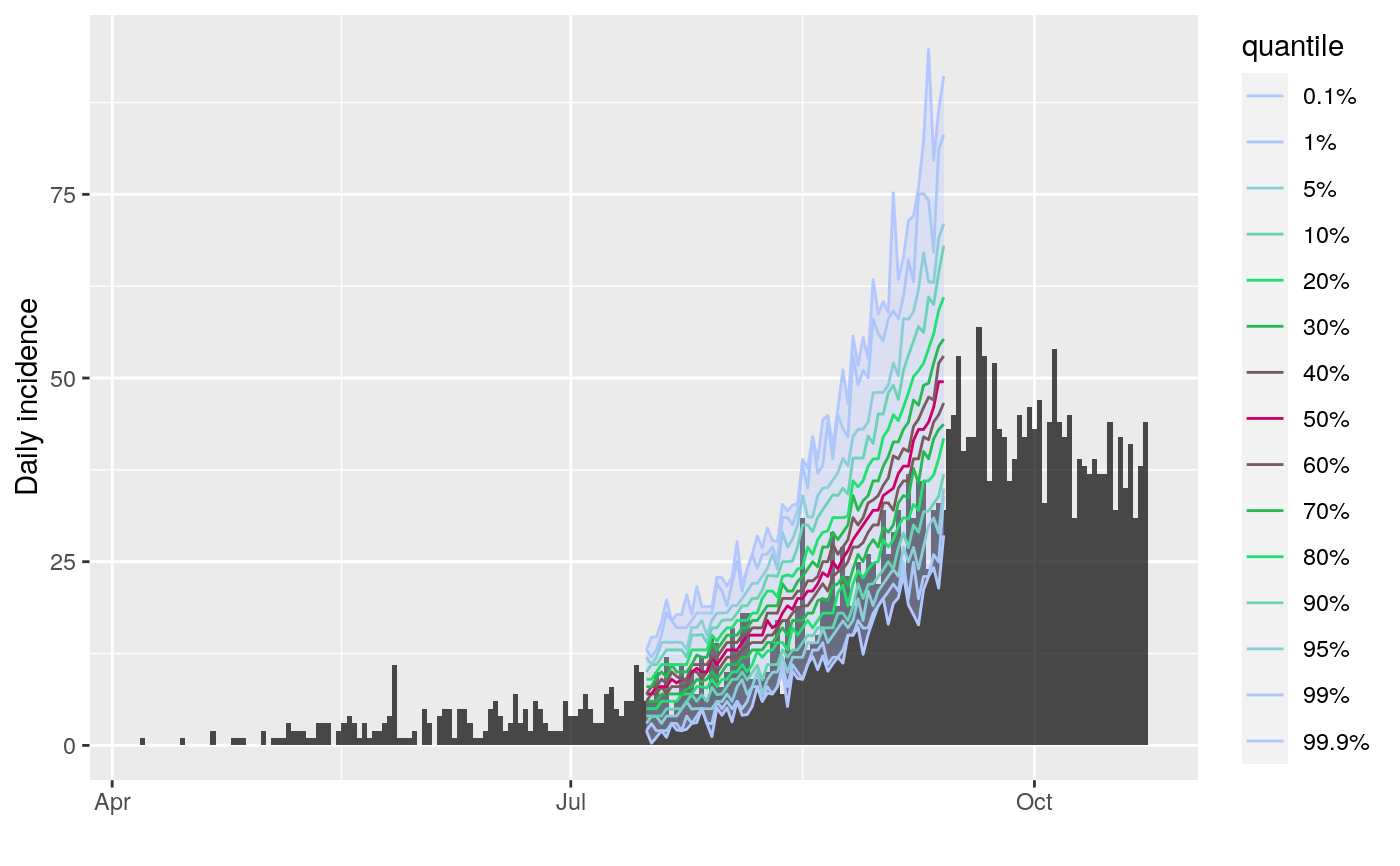The plot method of projections objects (output by the function project) shows quantiles of predicted incidence over time. The function add_projections can be used to add a similar plot to an existing incidence plot. This latter function is piping friendly (see examples).

# S3 method for projections
plot(x, ylab = NULL, title = NULL, ...)

add_projections(
p,
x,
quantiles = c(0.01, 0.05, 0.1, 0.5),
ribbon = TRUE,
boxplots = FALSE,
palette = quantile_pal,
quantiles_alpha = 1,
linetype = 1,
linesize = 0.5,
ribbon_quantiles = NULL,
ribbon_color = NULL,
ribbon_alpha = 0.3,
boxplots_color = "#47476b",
boxplots_fill = "grey",
boxplots_alpha = 0.8,
outliers = TRUE
)

## Arguments

x A projections object. An optional label for the y-axis. If missing will default to "predicted incidence" or, if cumulative, "predicted cumulative incidence" An optional title. Further arguments to be passed to add_projections. A previous incidence plot to which projections should be added. A vector of quantiles to plot, automatically completed to be symmetric around the median. A logical indicating if a ribbon should be drawn; defaults to TRUE. A logical indicating if boxplots should be drawn. A color palette to be used for plotting the quantile lines; defaults to quantile_pal. A number used to control the transparency of the quantile lines, from 0 (full transparency) to 1 (full opacity); defaults to 1. An integer indicating the type of line used for plotting the quantiles; defaults to 1 for a plain line. An integer indicating the size of line used for plotting the quantiles; defaults to 0.5. A vector of 2 quantiles to be used to determine the limits of the ribbon; if NULL (default); uses the most extreme quantiles if available; if quantiles are not provided, the daily range will be used. Any valid color, used for the ribbon. A number used to control the transparency of the ribbon, from 0 (full transparency) to 1 (full opacity); defaults to 0.3. Any valid color, used for the boxplot. Any valid color, used for filling the boxplot. A number used to control the transparency of the boxplots, from 0 (full transparency) to 1 (full opacity); defaults to 0.8. A logical indicating if outliers should be displayed alongside the boxplots; defaults to TRUE.

## See also

project to generate projections

## Examples


if (require(outbreaks) &&
require(distcrete) &&
require(incidence) &&
require(magrittr)) {

si <- distcrete("gamma",
interval = 1L,
shape = 2.4,
scale = 4.7,
w = 0.5)

i <- incidence(ebola_sim$linelist$date_of_onset)
plot(i)

## add projections after the first 100 days, over 60 days
set.seed(1)
proj <- project(x = i[1:100], R = 1.4, si = si, n_days = 60)

## plotting projections: different options
plot(proj)
plot(proj, quantiles = c(.025, .5)) # 95% CI
plot(proj, ribbon_color = "red", quantiles = FALSE) # range
plot(proj, ribbon_color = "red", quantiles = FALSE,
ribbon_quantiles = c(.025, .5))
plot(proj, boxplots = TRUE, quantiles = FALSE, ribbon = FALSE)
plot(proj, boxplots = TRUE, quantiles = FALSE, outliers = FALSE)
plot(proj, linetype = 3)

## adding them to incidence plot
plot(i) %>% add_projections(proj)
plot(i[1:160]) %>% add_projections(proj)
plot(i[1:160]) %>% add_projections(proj, boxplots = FALSE)
plot(i[1:160]) %>%
add_projections(proj, boxplots_alpha = .3, boxplots_color = "red")

## same, with customised quantiles and colors
quantiles <- c(.001, .01, 0.05, .1, .2, .3, .4, .5)
pal <- colorRampPalette(c("#b3c6ff", "#00e64d", "#cc0066"))
plot(i[1:200]) %>%
add_projections(proj, quantiles, palette = pal)

}#> Loading required package: outbreaks#> Loading required package: magrittr#> Scale for 'x' is already present. Adding another scale for 'x', which will
#> replace the existing scale.#> Scale for 'x' is already present. Adding another scale for 'x', which will
#> replace the existing scale.#> Scale for 'x' is already present. Adding another scale for 'x', which will
#> replace the existing scale.#> Scale for 'x' is already present. Adding another scale for 'x', which will
#> replace the existing scale.#> Scale for 'x' is already present. Adding another scale for 'x', which will
#> replace the existing scale.